Author: Oscar Cronquist Article last updated on February 01, 2019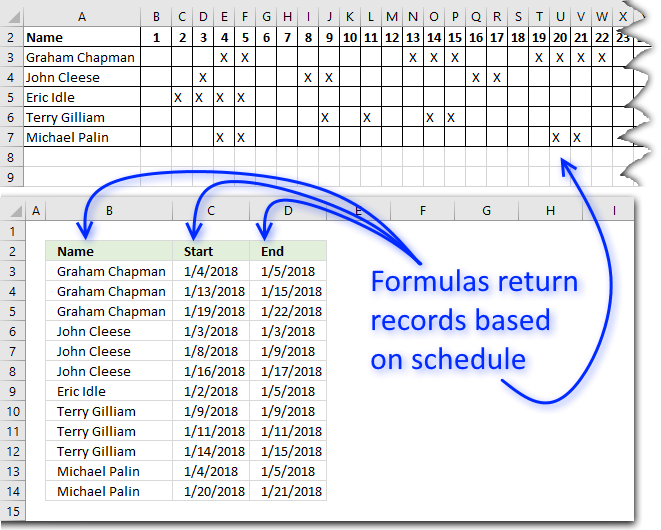The above picture shows you two formulas that extract names (column B) and date ranges (column C and D) based on a cross-reference schedule.

The schedule has dates in row 2, however, the cell is formatted to show only the day number.

Names are in column A and there are no duplicate names. An X indicates that a date is selected for the given person, multiple x's in a sequence is a date range.

The array formula in cell B3 returns the correct number names needed to return all date ranges:

=IFNA(INDEX(Sheet1!\$A\$3:\$A\$7, MATCH(FALSE, COUNTIF(\$B\$2:B2, Sheet1!\$A\$3:\$A\$7)=(MMULT((Sheet1!\$B\$3:\$AA\$7<>Sheet1!\$C\$3:\$AB\$7)*1, TRANSPOSE(COLUMN(Sheet1!\$B\$3:\$AA\$4)^0))/2), 0)), "")

Array formula in cell C3:

=SMALL(IF((Sheet1!\$B\$3:\$NB\$7<>Sheet1!\$C\$3:\$NC\$7)*(\$B3=Sheet1!\$A\$3:\$A\$7), Sheet1!\$B\$2:\$NB\$2+1, ""), COUNTIF(\$B\$3:B3, B3)*2-1)

Array formula in cell D3:

=SMALL(IF((Sheet1!\$B\$3:\$NB\$7<>Sheet1!\$C\$3:\$NC\$7)*(\$B3=Sheet1!\$A\$3:\$A\$7), Sheet1!\$B\$2:\$NB\$2, ""), COUNTIF(\$B\$3:B3, B3)*2)
1. Sheet1!\$A\$3:\$A\$7 is the cell reference to the names in the schedule.
2. Sheet1!\$B\$3:\$AA\$7 and Sheet1!\$C\$3:\$AB\$7 are a cell reference to the X's in the schedule.
Two are needed for the formula to count the number of date ranges.
Note! The cell refs have the same size, however, the latter is offset by one column.

To enter an array formula, type the formula in cell B3 then press and hold CTRL + SHIFT simultaneously, now press Enter once. Release all keys.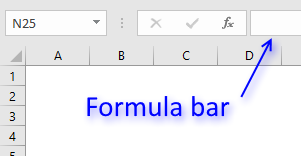The formula bar now shows the formula with a beginning and ending curly bracket telling you that you entered the formula successfully.

Don't enter the curly brackets yourself, they appear automatically.

### Explaining the formula in cell B3

Use "Evaluate Formula" on tab "Formula" on the ribbon to go through the steps.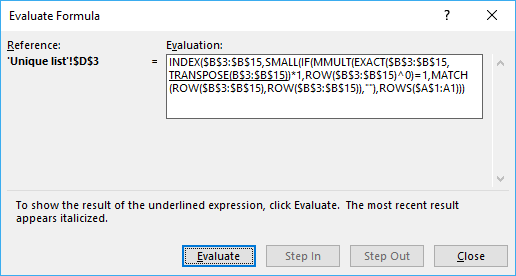#### Step 1 - Count previous values

COUNTIF(\$B\$2:B2,Sheet1!\$A\$3:\$A\$7)

becomes

COUNTIF("Name", {"Graham Chapman"; "John Cleese"; "Eric Idle"; "Terry Gilliam"; "Michael Palin"})

and returns {0;0;0;0;0}. None of the names have been displayed in cells above, remember that I am right now showing the calculation steps in cell B3.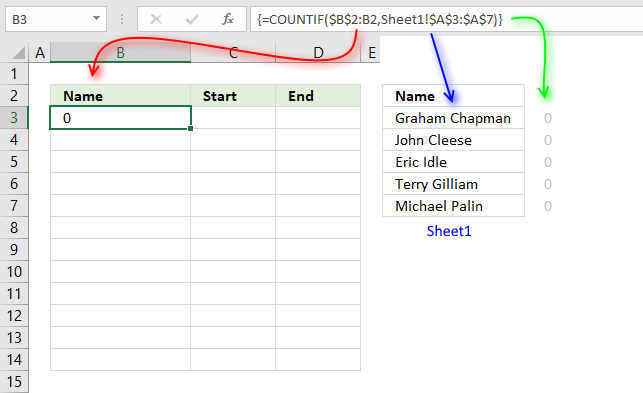As soon as the first name has been displayed the same number of times as it has date ranges the formula continues to the second name.

We are now going to count how many date ranges there is in the schedule per row.

#### Step 2 - Calculate where a cell value changes in a row

To count date ranges the formula needs to compare a cell with the next. To be able to compare all values in one calculation I use two cell ranges. The last cell range has the same size as the first, however, it is offset one column to the right.

(Sheet1!\$B\$3:\$AA\$7<>Sheet1!\$C\$3:\$AB\$7)*1

becomes

{0, 0, 1, 0, 1, 0, 0, 0, 0, 0, 0, 1, 0, 0, 1, 0, 0, 1, 0, 0, 0, 1, 0, 0, 0, 0;0, 1, 1, 0, 0, 0, ... , 0}

Part of the array is displayed in cell range B8:X12 in picture below.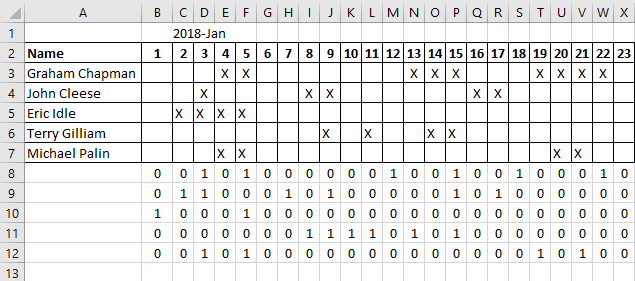#### Step 3 - Sum values per row

The amazing MMULT function allows you to sum numbers in an array per row or column. The earlier calculation step created an array that shows when a cell has a different value compared to the next cell.

That makes the array show when a date range starts and ends, to be able to count date ranges we must divide the sum of each row with 2.

(MMULT((Sheet1!\$B\$3:\$AA\$7 <> Sheet1!\$C\$3:\$AB\$7)*1, TRANSPOSE(COLUMN(Sheet1!\$B\$3:\$AA\$4)^0))/2)

becomes

({6; 6; 2; 6; 4}/2)

and returns {3; 3; 1; 3; 2}.

The array tells us that the first name has three date ranges, the second name has three date ranges and so on.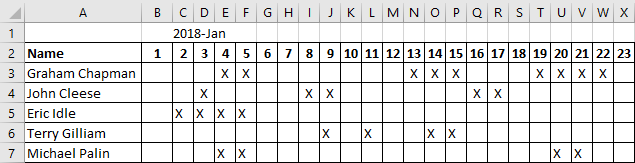The image above shows that the first name has three date ranges, the second has 3, the third has 1, the fourth has 3, and the last name has 2 date ranges.

The calculation is correct.

#### Step 4 - Compare arrays

The number of names displayed must match the corresponding number of date ranges.

To do that I compare the arrays, if they are equal the logical expression returns TRUE. If not equal it returns FALSE.

COUNTIF(\$B\$2:B2, Sheet1!\$A\$3:\$A\$7)=(MMULT((Sheet1!\$B\$3:\$AA\$7<>Sheet1!\$C\$3:\$AB\$7)*1, TRANSPOSE(COLUMN(Sheet1!\$B\$3:\$AA\$4)^0))/2)

{0;0;0;0;0}={3; 3; 1; 3; 2}

and returns {FALSE; FALSE; FALSE; FALSE; FALSE}.

#### Step 5 - Find position of first name that is shown less times than there is corresponding date ranges

The MATCH function allows you to identify the position of a specific value in an array or cell range, if the third argument in the MATCH function is 0 (zero) meaning EXACT match.

MATCH(FALSE, COUNTIF(\$B\$2:B2, Sheet1!\$A\$3:\$A\$7)=(MMULT((Sheet1!\$B\$3:\$AA\$7<>Sheet1!\$C\$3:\$AB\$7)*1, TRANSPOSE(COLUMN(Sheet1!\$B\$3:\$AA\$4)^0))/2), 0)

MATCH(FALSE, {FALSE; FALSE; FALSE; FALSE; FALSE}, 0)

and returns 1.

#### Step 6 - Get the name

The INDEX function gets the first value in cell range Sheet1!\$A\$3:\$A\$7.

INDEX(Sheet1!\$A\$3:\$A\$7, MATCH(FALSE, COUNTIF(\$B\$2:B2, Sheet1!\$A\$3:\$A\$7)=(MMULT((Sheet1!\$B\$3:\$AA\$7<>Sheet1!\$C\$3:\$AB\$7)*1, TRANSPOSE(COLUMN(Sheet1!\$B\$3:\$AA\$4)^0))/2), 0))

becomes

becomes

INDEX({"Graham Chapman"; "John Cleese"; "Eric Idle"; "Terry Gilliam"; "Michael Palin"}, 1)

and returns "Graham Chapman" in cell B3.

### Download Excel *.xlsx file

Generate records from matrix.xlsx